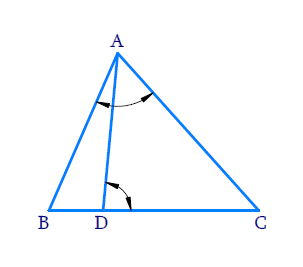# Ex.6.3 Q13 Triangles Solution - NCERT Maths Class 10

Go back to  'Ex.6.3'

## Question

$$D$$ is a point on the side $$BC$$ of a $$\Delta ABC$$ such that $$\angle ADC$$ $$=$$ $$\angle BAC$$. Show that $$\,C{{A}^{2}}=CB.CD$$ .

DiagramVideo Solution
Triangles
Ex 6.3 | Question 13

## Text Solution

Reasoning:

As we know if two triangles are similar then their corresponding sides are proportional.

Steps:

In $$\Delta ABC$$ and $$\Delta DAC$$

\begin{align} & \angle BAC=\angle ADC \\ & \text{(Given in the statement)}\\\\ & \angle ACB=\angle ACD\\ & \text{(Common angles)} \\\\ \Rightarrow \quad&\Delta ABC \sim \Delta DAC \\ & \text{(AA criterion)} \end{align}

If two triangles are similar,then their corresponding sides are proportional

\begin{align} \Rightarrow \quad\frac{C A}{C D}&=\frac{C B}{C A}\\\Rightarrow \quad C A^{2}&=C B \cdot C D\end{align}

Learn from the best math teachers and top your exams

• Live one on one classroom and doubt clearing
• Practice worksheets in and after class for conceptual clarity
• Personalized curriculum to keep up with school